﻿ Really Short Note on Examples of S-convex FunctionsModern International Journal of Pure and Applied Mathematics

2018;  2(1): 1-4

doi:10.5923/j.mijpam.20180201.01### Really Short Note on Examples of S-convex Functions

M. R. Pinheiro

IICSE University, USA

Correspondence to: M. R. Pinheiro, IICSE University, USA.
 Email: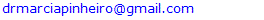Abstract

In this note, we present a few more important scientific remarks regarding the S-convexity phenomenon. This time, we talk about examples. That was one of the first queries Professor Mark Nelson had for us at the ANZIAM meeting that happened this year, in 2017, at the Wollongong University, Information Sciences building. We here talk about a very trivial example. Yet this example will prove a few really old results to be equivocated.

Keywords: Noindent Analysis, S-convexity, Example

Cite this paper: M. R. Pinheiro, Really Short Note on Examples of S-convex Functions, Modern International Journal of Pure and Applied Mathematics, Vol. 2 No. 1, 2018, pp. 1-4. doi: 10.5923/j.mijpam.20180201.01.

1. Introduction
2. Development
3. Conclusions
Note

### 1. Introduction

We have just presented the new definition for the phenomenon S-convexity at the ANZIAM meeting. Our talk was named Time to Think of S-convexity . The new definition is:
Definition 1. A function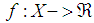, where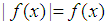, is told to belong to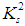if the inequality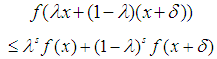holds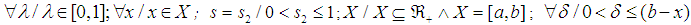.
Definition 2. A function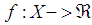, where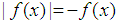, is told to belong to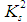if the inequality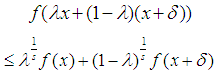holds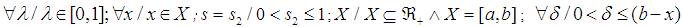.
Remark 1. If the inequality is obeyed in the supplementary1 situation by f , then f is said to be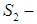concave.
This definition includes the essence of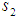-convexity as presented by Hudzik and Maligranda .
In this really short note, we will be proving that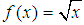belongs to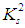as we now define it, and, with this, we will be proving that some of the claims from  are unfounded.

### 2. Development

Assume that it is not the case that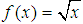is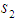-convex. That means that it is possible to find at least one value for a or b or even for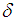or x such that it is not true that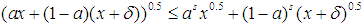. In this case, we know that (A)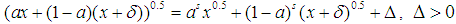.
a can always assume the value 0. If we replace a with 0 in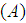, however, we get that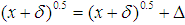. The last equation implies that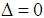, but this is a contradiction with our assumptions and therefore proves that our assumptions are equivocated. That leads us to the desired result, since the mistake was assuming thatwas not part of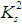.
The extract we see below this line came from .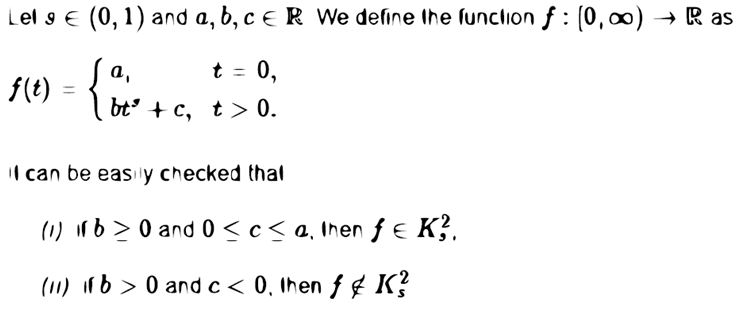Figure 1. Hudzik and Maligranda's theorem to generate examples
We can tell, from the graphs below, that moving one unit down won't make the graph escape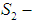convexity: It is just a matter of saying that x is now greater than 1. In this case,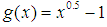does belong to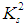, but, according to the Figure 1, that could not be true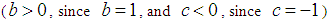.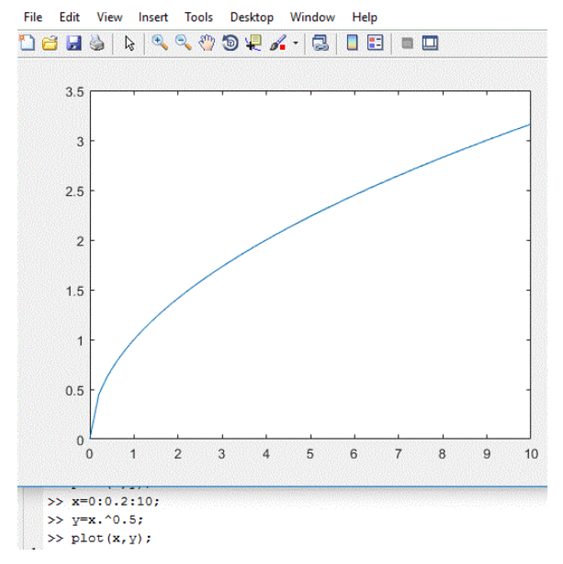MATLAB,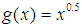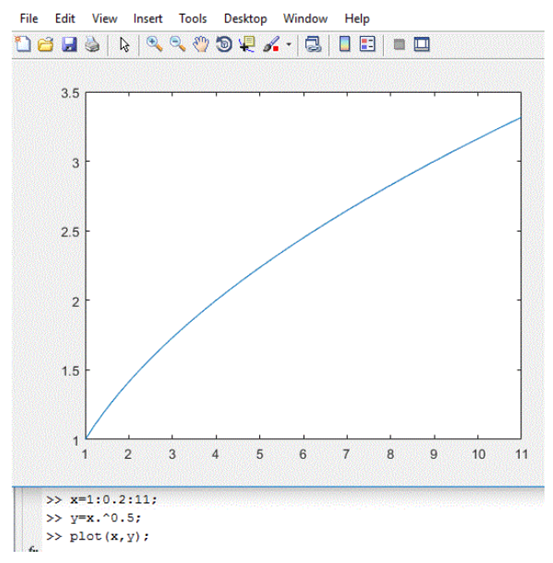MATLAB,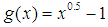One could allege that  talks about the domain starting from zero, and, in our counter-example, it would start from one. In this case, consider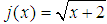.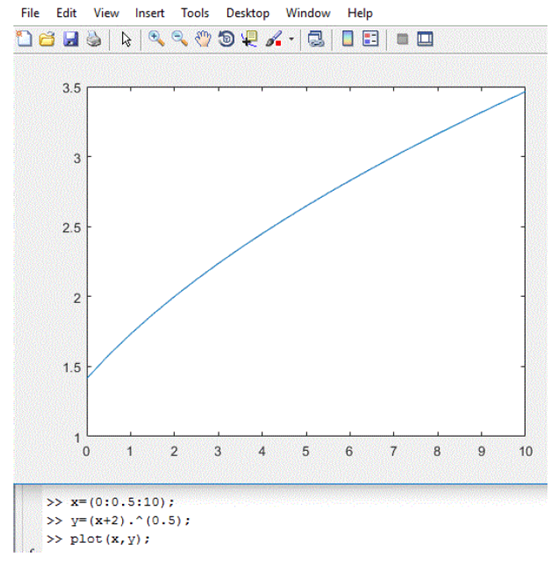Figure 4.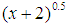The proof that it is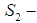convex is analogous. See: Assume that it is not the case that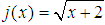is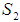-convex. That means that it is possible to find at least one value for a or b or even for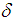or x such that it is not true that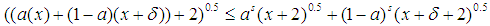. In this case, we know that (A)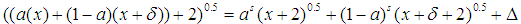,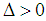. a can always assume the value 0. If we replace a with 0 in (A), however, we get that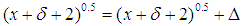. The last equation implies that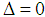, but this is a contradiction with our assumptions, and therefore proves that our assumptions are equivocated. That leads us to the desired result, since the mistake was assuming thatwas not part of.

### 3. Conclusions

In this paper, we have provided a very trivial example of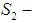convex function. In fact, we provided a family of trivial examples ofconvex functions (change the constant, values above and below 0). That should make Professor Mark Nelson like our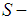convexity more, but it should also make Hudzik, Maligranda, Dragomir, and a few other professors a bit more upset, since we have proven that one of the theorems of Hudzik, supposed to generateconvex functions, cannot really be trusted. We have provided an entire family of trivial counter-examples to it here. Now the definition ofconvexity is settled through , so that we can write more about examples. Next, we will be analysing the work already done in terms of examples.

### Note

1. Supplementary here means '>', not '≥'.

### References

  M. R. Pinheiro. (2017). Time to Think about S-convexity. Retrieved from https://drmarciapinheiro.wordpress.com/2017/11/21/time-to-think-about-s-convexity/ on the 1st of December of 2017.  Hudzik, H. and Maligranda, L. (1994). Some Remarks on s-Convex Functions. Aequationes Mathematicae, 48, pp. 100-111.  Ozdemir, M. E., Yildiz, C., Akdemir, A. O., and Set, E. (2013). On Some Inequalities for S-convex Functions and Applications. Journal of Inequalities and Applications.  M. R. Pinheiro. (2017). Second Note on the New Shape of S-convexity. Modern International Journal of Pure and Applied Mathematics, 1(3), pp. 46-49.